Theory of Augmented Quantum Reality | OMICS International
Journal of Lasers, Optics & Photonics

# Theory of Augmented Quantum Reality

Solomon Budnik*

UTG-PRI TD, Tel Aviv, Israel

*Corresponding Author:
Solomon Budnik
President, UTG-PRI TD
Tel Aviv, Israel
E-mail: [email protected]

Received Date: June 28, 2016 Accepted Date: July 13, 2016 Published Date: July 14, 2016

Citation: Budnik S (2016) Theory of Augmented Quantum Reality. J Laser Opt Photonics 3: 133. doi: 10.4172/2469-410X.1000133

Copyright: © 2016 Budnik S. This is an open-access article distributed under the terms of the Creative Commons Attribution License, which permits unrestricted use, distribution, and reproduction in any medium, provided the original author and source are credited.

Visit for more related articles at Journal of Lasers, Optics & Photonics

#### Abstract

We present here our quantum propagation and entanglement system for virtual reality remote space computers and TVs with photonic displays in laser activated imagery. This concept is based on 1935 Einstein-Podolsky-Rosen Argument in Quantum Theory. Einstein maintains “the interpretation, according to which |ψ|² expresses the probability that this particle is found at a given point, assumes an entirely peculiar mechanism of action at a distance, which prevents the wave continuously distributed in space from producing an action in two places on the screen. Einstein continues: “in my opinion, one can remove this objection only in the following way, that one does not describe the process solely by the Schrödinger wave, but that at the same time one localizes the particle during propagation

#### Keywords

Quantum; Computers; Photonic displays; Computers; TV

#### Introduction

With applied Einstein’s concept to our 3D quantum phase space display system (see image below) in which all possible states of a system are represented, with each possible state of the system corresponding to one unique point in the phase space of all possible values of position and momentum variables, where every degree of freedom or parameter of the system is represented as an axis of a multidimensional space. The concept of phase space was developed in the late 19th century by Ludwig Boltzmann, Henri Poincaré, and Willard Gibbs (Figures 1 and 2). Equations for harmonic oscillator in 3D spherical coordinates of our 3D quantum phase space display system are in our separate paper. This allows us to get generic boundary conditions for the quantum oscillator on N dimensional complex projective space (CPN) and on its noncompact version i.e., Lobachewski space (LN) in presence of constant magnetic field. As a result, we get a family of energy spectrums for the oscillator. Motion of a classical particle in 3-dimensional Lobachevsky and Riemann spaces is studied in the presence of an external magnetic field which is analogous to a constant uniform magnetic field in Euclidean space. In both cases three integrals of motions are constructed and equations of motion are solved exactly in the special cylindrical coordinates on the base of the method of separation of variables. In Lobachevsky space there exist trajectories of two types, finite and infinite in radial variable; in Riemann space all motions are finite and periodical. The invariance of the uniform magnetic field in tensor description and gauge invariance of corresponding 4-potential description is demonstrated explicitly. The role of the symmetry is clarified in classification of all possible solutions, based on the geometric symmetry group, SO(3,1) and SO(4) respectively.

#### Elaboration

Our quantum harmonics electronic system will be based on a quantum carrier (quantum ball or spehere) and jump-resonance phenomena of nonlinear feedback control systems. Second harmonic generation (see below) with resonant enhancement is applicable to our quantum space display model discussed here (Figure 3). The nonlinearities are those whose outputs are single-valued odd functions of the inputs and are independent of frequencies of the photonic inputs. The general conditions under which jump-resonance occurs will be given and the system with saturation nonlinearity will be analysed. The essential objective is to define the contours on the complex plane for the constant values of system variables, e.g., input amplitude, amplitude ratio, and phase shift. Common Frequency Hopping Spread Spectrum (FHSS) will be upgraded by us in our quantum harmonics system to randomly propagate atomic particles by photonic ally switching from one signal carrier (quantum tube, see our quantum harmonics paper) to other quantum channels in thereby achieved dynamic equilibrium beyond chaotic interference. Fermi-Dirac distribution function [1,2] and (electro vacuum) solutions of the Einstein (Einstein-Maxwell) field equations are applicable. Same concept can be applied in our remote quantum loops space display system (see the diagram in Figure 4 below) to constitute space computes and TVs (Figure 5). We accordingly introduce here the notions of a spinning quantum spring (Figure 5) to constitute a spinning quantum ball or sphere created by a multimodal quantum structure in Figure 4. It appears that our quantum ball carrier is a continuum of possible energies. When the carrier is confined to a 3D space, the quantum energy levels begin to spread out and the quantum nature becomes detectable, i.e., electrons will settle in the quantum ball and not in the adjacent layers. This carrier will then exhibit the quantum effects imposed on it, where the number of particles trapped in the carrier can be controlled by an external voltage. Compare our multimodal quantum loops 3D diagram in Figure 4 with Feynman diagram of quantum field geometry (Figure 6): Feynman diagram quantum field geometry To visualize the spinning nature of our multimodal quantum system in Figure 4, we show in Figure 5 the spinning central quantum spring, which sustains our quantum ball’s overall structure (Figures 6-12).

Figure 3: Cartoon depicting ordered molecules at a small spherical surface. An ultrafast pump laser pumps light with frequency ω which generates light at 2ω from the locally non-centro symmetric media.

Figure 4: This diagram shows integrated quantum loops with central spinning quantum spring, which defines the horizon of the rotating plain constituting a quantum carrier-quantum ball to create a 3D space plasma imagery display in self-generated and contained e.m. field as in a ball lighting to be activated by a tunable pulse laser via spectral prism and acoustic membrane in a combination of colours and sounds of a quantum computer and TV.

Figure 6: Spinning quantum spring to create the quantum ball or sphere (compare with the ball lighting).

Figure 9: shows our remote spinning quantum spiral in 3D space display per classical field theory with space-time manifold M (figure 4 below) and field space F, where ?: M F, and action critical points are S[?], dS=0.

Figure 11: Compare our 3D spinning quantum ball display in figure 3 with the pictured here in ball lightning structure.

#### Electron-ionic Model of Ball Lightning

From wikiversity

The electron-ionic model of ball lightning was represented by Sergey G. Fedosin, a physicist and the philosopher from Perm, Russia, and Kim, from Perm state university, in a number of works. In this model, ball lightning is a cluster of the very hot ionized air with the positive charge in general, whose shell consists of the rapidly revolving electrons with the total current up to 1,4•105 A. Ball lightning as whole is supported by the balance of the electromagnetic forces, which act between the charges. Positive ions inside the lightning are distributed freely as a result of the spherical symmetry, and attract to themselves the electrons of shell, retaining them from the dispersion. According to the model, the ball lightning is formed from two close branches of a linear lightning at the time of termination of current in the main channel with the subsequent closure of branches in a current ring (Figure 13). Equatorial cross-section model of ball lightning as a distinct ring on the current sheet spheroidal shape. R-radius of rotation of ions in the equilibrium shell around the magnetic field with induction B, r-radius of the outer electron shell. Electronic currents in the shell create strong magnetic field inside the lightning. These currents are perpendicular to rotational axis, the diameter of rotation decreases to the poles, where magnetic field grows. This retains positive ions from the dispersion along the rotational axis due to the effect of magnetic bottle. Basic magnetic field inside the lightning is directed along the rotational axis. I.e., ions can move along the axis along the lines of magnetic field. From other side, the ions revolve in the circle perpendicularly to axis under the action of Lorentz force with respect to their thermal velocity .

Figure 13: Equatorial cross-section model of ball lightning as a distinct ring on the current sheet spheroidal shape.

As a result at a certain distance from the axis of lightning appears the intersection of two ion flows, which is observed as the luminous shells inside the lightning. Emission from the shells appears from friction and recombination of the being intersected ion flows. Theory predicts from the first principles the maximum diameter of ball lightning 34 cm. With the larger size the summary charge of lightning, which has positive sign, grows to the value of 10 –5 C and appears the electrical breakdown of air near the lightning. The energy of the lightning in this case reaches 10.6 kJ, the current in the shell 1.4?10 5 A, the internal magnetic field of 0.5

Tesla. Because of its charge ball lightning does not simply float under the action of the force of Archimedes, but it is retained by electric force from clouds and the induced charge on the Earth . The formula for the maximum radius of ball lightning has the form: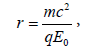#### Derivation of the Fermi-Dirac distribution function

We start from a series of possible energies, labeled Ei. At each energy we can have gi possible states and the number of states that are occupied equals gifi, where fi is the probability of occupying a state at energy Ei. The number of possible ways - called configurations - to fit gi fi electrons in gi states, given the restriction that only one electron can occupy each state, equals: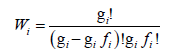This equation is obtained by numbering the individual states and exchanging the states rather than the electrons. This yields a total number of gi! possible configurations. However since the empty states are all identical, we need to divide by the number of permutations between the empty states, as all permutations cannot be distinguished and can therefore only be counted once . In addition, all the filled states are indistinguishable from each other, so we need to divide also by all permutations between the filled states, namely gifi!

The number of possible ways to fit the electrons in the number of available states is called the multiplicity function. The multiplicity function for the whole system is the product of the multiplicity functions for each energy Ei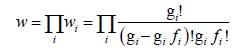Using Stirling’s approximation, one can eliminate the factorial signs, yielding: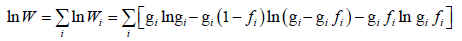The total number of electrons in the system equals N and the total energy of those N electrons equals E. These system parameters are related to the number of states at each energy, gi, and the probability of occupancy of each state, fi, by: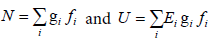According to the basic assumption of statistical thermodynamics, all possible configurations are equally probable. The multiplicity function provides the number of configurations for a specific set of occupancy probabilities, fi. The multiplicity function sharply peaks at the thermal equilibrium distribution. The occupancy probability in thermal equilibrium is therefore obtained by finding the maximum of the multiplicity function, W, while keeping the total energy and the number of electrons constant.

For convenience, we maximize the logarithm of the multiplicity function instead of the multiplicity function itself. According to the Lagrange method of undetermined multipliers, we must maximize the following function: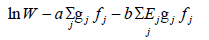Where a and b need to be determined. The maximum multiplicity function is obtained from: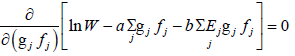Which can be solved, yielding: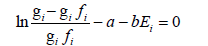or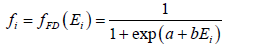Which can be written in the following form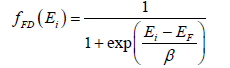With Ei=1/b and EF=-a/b. The symbol EF was chosen since this constant has units of energy and will be the constant associated with this probability distribution.

Taking the derivative of the total energy, one obtains: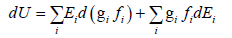Using the Lagrange equation, this can be rewritten as: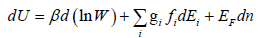Any variation of the energies, Ei, can only be caused by a change in volume, so that the middle term can be linked to a volume variation dV.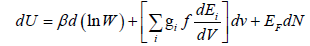This to the thermodynamic identity: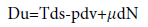One finds that βd=kT and S=k lnW . The energy, EF, equals the energy associated with the particles, Σ.

The comparison also identifies the entropy, S, as being the logarithm of the multiplicity function, W, multiplied with Boltzmann’s constant.

The Fermi-Dirac distribution function then becomes: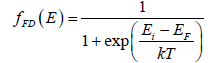#### References

Select your language of interest to view the total content in your interested language

### Article Usage

• Total views: 1243
• [From(publication date):
December-2016 - Dec 05, 2019]
• Breakdown by view type
• HTML page views : 1140Can't read the image? click here to refresh